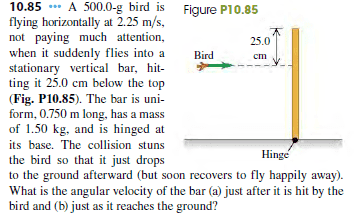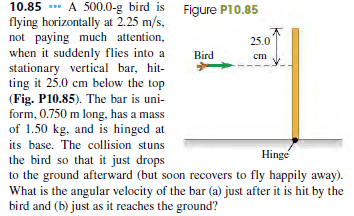# Rotational Motion - Conservation angular momentum

## Homework Statement

A 500.0-g bird is flying horizontally at 2.25 m>s, not paying much attention, when it suddenly flies into a stationary vertical bar, hitting it 25.0 cm below the top(Fig. P10.85). The bar is uniform, 0.750 m long, has a mass of 1.50 kg, and is hinged at its base. The collision stuns the bird so that it just drops to the ground afterward (but soon recovers to fly happily away).What is the angular velocity of the bar (a) just after it is hit by the bird and (b) just as it reaches the ground?

## Homework Equations

L = Iw
L = p x r
I = ML^2/3 for a rod

## The Attempt at a Solution

a) The angular momentum before the collision:
L1 = Lbird + Lrod = p x r + 0 = mvr
Then afterwards:
L2 = Lbird + Lrod = 0 + Iw

we get the equation: mvr = Iw from conservation of momentum

Plug in the numbers: 0.5 * 2.25 * (0.75-0.25) = 1.5 * (0.75)^2 * w/3

Solve for w = 2 rads/s

b) I'm not really sure how to attack this one, the angular momentum won't be conserved because of the gravity enacting a torque on the object.

Any suggestions?

#### Attachments

•Physics problem 2.png
16 KB · Views: 744

SammyS
Staff Emeritus
Homework Helper
Gold Member

## Homework Statement

A 500.0-g bird is flying horizontally at 2.25 m>s, not paying much attention, when it suddenly flies into a stationary vertical bar, hitting it 25.0 cm below the top(Fig. P10.85). The bar is uniform, 0.750 m long, has a mass of 1.50 kg, and is hinged at its base. The collision stuns the bird so that it just drops to the ground afterward (but soon recovers to fly happily away).What is the angular velocity of the bar (a) just after it is hit by the bird and (b) just as it reaches the ground?

## Homework Equations

L = Iw
L = p x r
I = ML^2/3 for a rod

## The Attempt at a Solution

a) The angular momentum before the collision:
L1 = Lbird + Lrod = p x r + 0 = mvr
Then afterwards:
L2 = Lbird + Lrod = 0 + Iw

we get the equation: mvr = Iw from conservation of momentum

Plug in the numbers: 0.5 * 2.25 * (0.75-0.25) = 1.5 * (0.75)^2 * w/3

Solve for w = 2 rads/s

b) I'm not really sure how to attack this one, the angular momentum won't be conserved because of the gravity enacting a torque on the object.

Any suggestions?You might consider using conservation of energy for part (b).

•HoodedFreakYou might consider using conservation of energy for part (b).

Okay, so if I say 1/2Mv1^2 + MgL/2 = 1/2Mv2

where v = wR

Then, we get w1*L/2 + gL = w2*L/2

THen 2 + 2g = w2

SO we get 2 + 2g = w2

And finally we get w2 = 21.62

Which isn't the right answer, where did I go wrong?

gneill
Mentor
How do you justify the use of L/2 for the "radius" when calculating the initial KE?

Instead, use the angular form for the KE: ##\frac{1}{2} I \omega^2##

•HoodedFreak
How do you justify the use of L/2 for the "radius" when calculating the initial KE?

Instead, use the angular form for the KE: ##\frac{1}{2} I \omega^2##

I was using the linear velocity of the center of mass. Isn't the angular kinetic energy for rotation about the center of mass, wouldn't this be zero in this case?
This is why I was so confused for this question.

I realised I made alot of mistakes on my previous post, so let me correct myself

1/2Mv12 + MgL/2 = 1/2Mv22

where v = wR, R = L/2 in this case

Then, we get 1/2 * (w1R)2 + gR = 1/2 * (w2R)2

So, 1/2 * 4 * R + g = 1/2 * w22*R

THen (2R + g)*2 / R = ww2

Solving for w, gives w = 7.5

haruspex
Homework Helper
Gold Member
2020 Award
I was using the linear velocity of the center of mass. Isn't the angular kinetic energy for rotation about the center of mass, wouldn't this be zero in this case?
This is why I was so confused for this question.
You can either use linear KE of the centre of mass and add angular KE about the mass centre (which is not zero, since it is rotating), or use angular kinetic energy about the hinge.

For part a), I'm not sure how to interpret "the bird drops to the ground". You seem to have read it as saying the bird loses all horizontal momentum, which would imply some degree of elastic collision. You might be reading too much into the wording. I think you should consider it a purely inelastic collision.

•HoodedFreak
You can either use linear KE of the centre of mass and add angular KE about the mass centre (which is not zero, since it is rotating), or use angular kinetic energy about the hinge.

For part a), I'm not sure how to interpret "the bird drops to the ground". You seem to have read it as saying the bird loses all horizontal momentum, which would imply some degree of elastic collision. You might be reading too much into the wording. I think you should consider it a purely inelastic collision.

Hmmm, well I got the answer right for part a) considering it as an elastic collision, if I considered it as an inelastic collision, then I would get:

L1 = 0.5*2.25*(0.75-0.25)
L2 = (ML^2/3 + 0.5*0.52)*w

Equating the two: 2.25/4 = (1.5*0.752/3 + 0.53) * w
which gives me an answer of w = 1.38 for the rod and bird, which is not the answer the book gives

SammyS
Staff Emeritus
Homework Helper
Gold Member
Hmmm, well I got the answer right for part a) considering it as an elastic collision, if I considered it as an inelastic collision, then I would get:

L1 = 0.5*2.25*(0.75-0.25)
L2 = (ML^2/3 + 0.5*0.52)*w

Equating the two: 2.25/4 = (1.5*0.752/3 + 0.53) * w
which gives me an answer of w = 1.38 for the rod and bird, which is not the answer the book gives
The wording of the problem is not too clear on this point, but I interpreted part (a) the way you did initially.

•HoodedFreak
haruspex
Homework Helper
Gold Member
2020 Award
Hmmm, well I got the answer right for part a) considering it as an elastic collision, if I considered it as an inelastic collision, then I would get:

L1 = 0.5*2.25*(0.75-0.25)
L2 = (ML^2/3 + 0.5*0.52)*w

Equating the two: 2.25/4 = (1.5*0.752/3 + 0.53) * w
which gives me an answer of w = 1.38 for the rod and bird, which is not the answer the book gives
ok.